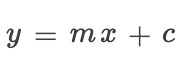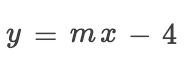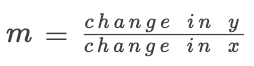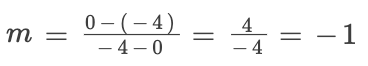### SAT数学：图像例题 5

2019-09-12 SAT-数学 阅读

# Rank: Easy Topic: Algebra, Equation of a line

Below is a question from a recent SAT exam. It is a non-calculator question that would typically take a student 1-2 minutes to complete.Which of the following is an equation of line ℓ in the
xy-plane above?

(A) x – y = –4
(B) x – y = 4
(C) x+ y = –4
(D) x+ y = 4

To begin, we must recall that the equation on a straight line comes in the formWhere m is the slope and c is the y-intercept.
We will start by finding the y-intercept. This is the point where the line crosses the y-axis.We can clearly see in this diagram that the line meet the y-axis at -4. So we can replace c in our equation.Now, we need to find the slope of the line. To find this we use the formula:So, we need to find to point on our line, any two will do but, I will pick the two points where it meets an axis.
(0,-4) and (-4,0)
So our two x-coordinates are 0 and -4 whilst our two y-coordinates are -4 and 0.
We can put these into the formula:Therefore,
m=-1
We put this now into our equation for our line.
y=-x-4
We then can move x to the LHS of the equation.
x+y=-4
And we can see that the answer is C.• 地址：401-5811 Cooney Rd, Richmond, BC
• 地址：3030-8171 Ackroyd Rd, Richmond, BC
• 客服热线：604-370-0579 & 604-370-2569
• 联系邮箱：service@forbest.com

• 地址：江苏省无锡市梁溪区清扬路268号三楼
• 客服热线：181-1861-8855

•官方公众号
•加拿大官方客服
•中国官方客服